# Measure power at Power Distribution Panel (PDP) with 240/120 VAC Split Phase Service

Hi,

What products do you recommend to measure the power coming into the power distribution panel for a 240/120 VAC Split Phase Service? I live in Canada.
I would like a 6 channel module to measure 3 voltages and 3 currents.
See attached image.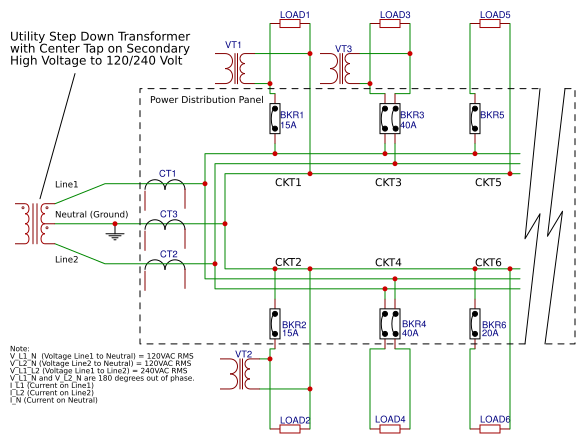For this type of panel, some suggest using 2 Current Transformers (CT1 and CT2) and 2 voltage transformers (VT1 and VT2), however that assumes the loads across L1-N and L2-N are equal. If the loads are not balanced, and that is probably 99% of the cases, then there will be some current flowing in N (Ground) back to the transformer. This is a source of error that can be eliminated with a 3rd CT and 3rd VT.

Do you have any products that could support 6 chanels for 3 CTs and 3 VTs ?

Thank you

-Mark

In the US, 120/240 is normally a split-phase.
I’ve seen 120/240 open delta’s, but that requires a 4-wire service.

Would this service be a corner grounded delta?
Is it truly a 2-wire service, or does the Neutral actually tie ?
I just don’t understand how you could have 120V without any reference to ground.
Again, just curious…

I updated the diagram because it wasn’t quite accurate.

The most common service in Canada is 240/120 VAC Split phase service, just like most of the USA.
It is not a corner grounded delta.

It is a 3-wire service; 2 main wires with a smaller 3rd wire as neutral (ground). The neutral wire is connected to the center tap of the transformer and the neutral bus bar of the power distribution panel. The neutral (ground) wire is also connected to a ground rod in the earth near the house. The neutral (ground) wire may be connected to a ground rod near the transformer, I am not 100% sure though.

-Mark

Hi Mark,

Looking at your schematic I see no reason for more than 2 voltage monitors. You need to measure between Neutral/L1 and Neutral/L2.

Now as for the CTs for monitoring amperage do you want to monitor current draw of all 6 loads individually or just the total current draw? If you just want to monitor the overall current draw of the system then you just need 2 CTs(one on L1 and one on L2). If you want to monitor the current draw of each load individually then you will need 6 CTs(one on each load).

My goal is to know the power consumed for some business servers running in my house.
Also, I updated the original drawing, for clarity.

Load Group 1 (Personal Use) = CKT1, CKT2, CKT6

My plan was to determine

• the power used for all circuits (total) in the distribution panel
• the power for each circuit in load group 2 (CKT3, CKT4, CKT5)
• the power used for all circuits (total) load group 1

After some more thinking and calculations, @Travis you are correct, I only need 2 VTs and 2 CTs.

The above schematic can be simplified as: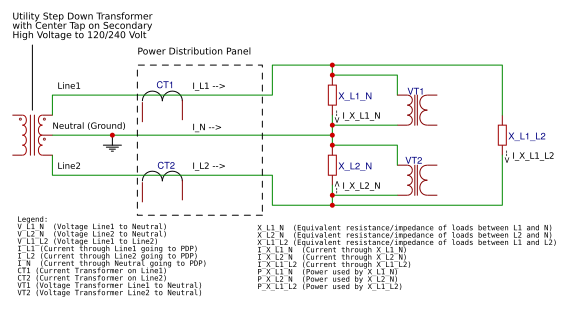Calculate total power going through PDP

``````P_PDP = P_X_L1_N
+ P_X_L2_N
+ P_X_L1_L2

P_PDP = V_L1_N * I_X_L1_N
+ V_L2_N * I_X_L2_N
+ V_L1_L2 * I_X_L1_L2
``````

Let

``````V_L1_N = 120 VAC RMS
V_L2_N = 120 VAC RMS
``````

Because V_L1_N and V_L2_N are 180 degrees out of phase
when V_L1_N is max (`120V*SQRT(2)=169.7V`), V_L2_N will be min (`-120V*SQRT(2)=-169.7V`)
therefore the RMS voltages can be added together.
Let

``````V_L1_L2 = V_L1_N + V_L2_N
V_L1_L2 = 240 VAC RMS
``````

Substitute `V_L1_L2` for `(V_L1_N + V_L2_N)`

``````P_PDP = V_L1_N * I_X_L1_N
+ V_L2_N * I_X_L2_N
+ (V_L1_N + V_L2_N) * I_X_L1_L2
``````

Multiply `(V_L1_N + V_L2_N)` by `I_X_L1_L2`

``````P_PDP = V_L1_N * I_X_L1_N
+ V_L2_N * I_X_L2_N
+ (V_L1_N * I_X_L1_L2) + (V_L2_N * I_X_L1_L2)
``````

Gather common terms for `V_L1_N` and `V_L2_N`

``````P_PDP = V_L1_N * (I_X_L1_N + I_X_L1_L2)
+ V_L2_N * (I_X_L2_N + I_X_L1_L2)
``````

From diagram, observe that

``````I_L1 = I_X_L1_N + I_X_L1_L2
I_L2 = I_X_L2_N + I_X_L1_L2
``````

Substitute 2 equations above into P_PDP equation

``````P_PDP = V_L1_N * (I_L1)
+ V_L2_N * (I_L2)
``````

Rewrite P_PDP equation using current and voltage transformers

``````P_PDP = V_VT1 * I_CT1
+ V_VT2 * I_CT2
``````

Only 2 VTs and 2 CTs required to calculate total power through the PDP.

So what happens if the loads in the PDP are not balanced between line1 and line2 ?
Answer: There will be current flowing through neutral wire (`I_N`), however the calculated power for the PDP will not be affected because the extra current flowing in the neutral will also be seen in either line1 or line2.

For example, if there is heavier load on line1, the differential current between line1 and line2 flows through the neutral wire to balance the circuit. This extra current also flows though line1 and will be observed by CT1, so the imbalance is captured perfectly.

`I_N` depends on ratio of `X_L1_N` to `X_L2_N`
`I_N = I_L1 + I_L2` (I_L1 and I_L2 point in opposite directions, so adding them will effectivly be the difference)

When `X_L1_N = X_L2_N`
then `I_N` is 0

When `X_L1_N < X_L2_N`
then `I_N` is Neg on first 180 degrees of waveform
and `I_N` is Pos on last 180 degrees of waveform

When `X_L1_N > X_L2_N`
then `I_N` is Pos on first 180 degrees of waveform
and `I_N` is Neg on last 180 degrees of waveform

So in summary, yes, only 2 VTs (VT1 and VT2) and 2 CTs (CT1 and CT2) are required to measure the total power flowing through the power distribution panel.

Measuring individual circuits/branches

When measuring the individual circuits, no new VTs are required. I do not need a VT between Line1 and Line2 because `V_L1_L2 = V_L1_N - V_L2_N`. So I know the 3 required voltages in the system.

I see an individual CT is required for each circuit I want to know the power consumption.

Product recommendations
So which product would you recomment to measure the 2 VTs and 6 CTs (2 CTs for the mains and 4 CT’s for some branch circuits) ?
I would prefer to use 1 board that has VT and CT measurments and does power calculations on the board. Doing VT measurement on one board and CT measurement on another and then doing the math in a raspberry PI, would provide a source of error, especially if power factor is far from 1.0.

Thank you,

-Mark

Also, here is what the waveforms would look like in 3 load scenaios: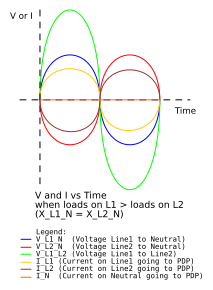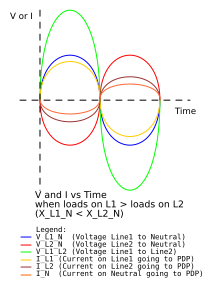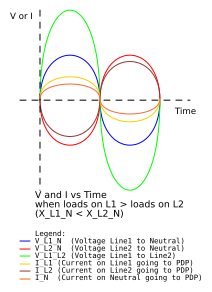And that’s what intrigued me with your Original Diagram that showed a 2-wire service with No Service Neutral. I thought you Canadians were using some Black Magic up there, and I wanted in-on-it.

But now your mentioning PT’s, which “here” normally means Power Transformer, typically used for High Side Metering. I assume you are referring to a Voltage (Potential) Transformer, a step-down for voltage measurement ?

Unless I’m missing something, you simply need a CT and Voltage reference for each Phase (2).

1 Like

Yeah no black magic where I live. We have a neutral wire.

However, I could imagine the system working without a neutral wire and using the earth as a neutral conductor. There are many electrical grids which use one wire plus the earth to distribute power. They are called single wire earth ground or SWEG systems. Those systems rely on the earth having enough conductivity to carry the current and would be affected by ground rod installation, moisture and temperature of the soil. I’m glad we have the third wire to eliminate the uncertainty of using earth as a conductor.

And Yes, when I said PT I did mean potential transformer not power transformer. My bad, I will correct that.

And yes, for my solution I need a VT and CT for each main line(leg) to get total power going to the PDP. And I need a CT for each branch circuit to know it’s power.